Regression-Discontinuity Analysis

# Regression-Discontinuity Analysis

## Analysis Requirements

The basic RD Design is a two-group pretest-posttest model as indicated in the design notation. As in other versions of this design structure (e.g., the Analysis of Covariance Randomized Experiment, the Nonequivalent Groups Design), we will need a statistical model that includes a term for the pretest, one for the posttest, and a dummy-coded variable to represent the program.

## Assumptions in the Analysis

It is important before discussing the specific analytic model to understand the assumptions which must be met. This presentation assumes that we are dealing with the basic RD design as described earlier. Variations in the design will be discussed later. There are five central assumptions which must be made in order for the analytic model which is presented to be appropriate, each of which is discussed in turn:

### 1. The Cutoff Criterion.

The cutoff criterion must be followed without exception. When there is misassignment relative to the cutoff value (unless it is known to be random), a selection threat arises and estimates of the effect of the program are likely to be biased. Misassignment relative to the cutoff, often termed a “fuzzy” RD design, introduces analytic complexities that are outside the scope of this discussion.

### 2. The Pre-Post Distribution.

It is assumed that the pre-post distribution is describable as a polynomial function. If the true pre-post relationship is logarithmic, exponential or some other function, the model given below is misspecified and estimates of the effect of the program are likely to be biased. Of course, if the data can be transformed to create a polynomial distribution prior to analysis the model below may be appropriate although it is likely to be more problematic to interpret. It is also sometimes the case that even if the true relationship is not polynomial, a sufficiently high-order polynomial will adequately account for whatever function exists. However, the analyst is not likely to know whether this is the case.

### 3.Comparison Group Pretest Variance.

There must be a sufficient number of pretest values in the comparison group to enable adequate estimation of the true relationship (i.e., pre-post regression line) for that group. It is usually desirable to have variability in the program group as well although this is not strictly required because one can project the comparison group line to a single point for the program group.

### 4. Continuous Pretest Distribution

Both groups must come from a single continuous pretest distribution with the division between groups determined by the cutoff. In some cases one might be able to find intact groups (e.g., two groups of patients from two different geographic locations) which serendipitously divide on some measure so as to imply some cutoff. Such naturally discontinuous groups must be used with caution because of the greater likelihood that if they differed naturally at the cutoff prior to the program such a difference could reflect a selection bias which could introduce natural pre-post discontinuities at that point.

### 5. Program Implementation

It is assumed that the program is uniformly delivered to all recipients, that is, that they all receive the same dosage, length of stay, amount of training, or whatever. If this is not the case, it is necessary to model explicitly the program as implemented, thus complicating the analysis somewhat.

## The Curvilinearity Problem

The major problem in analyzing data from the RD design is model misspecification. As will be shown below, when you misspecify the statistical model, you are likely to get biased estimates of the treatment effect. To introduce this idea, let’s begin by considering what happens if the data (i.e., the bivariate pre-post relationship) are curvilinear and we fit a straight-line model to the data.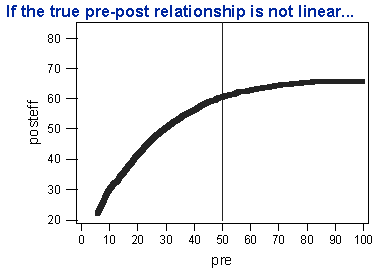Figure 1. A curvilinear relationship.

Figure 1 shows a simple curvilinear relationship. If the curved line in Figure 1 describes the pre-post relationship, then we need to take this into account in our statistical model. Notice that, although there is a cutoff value at 50 in the figure, there is no jump or discontinuity in the line at the cutoff. This indicates that there is no effect of the treatment.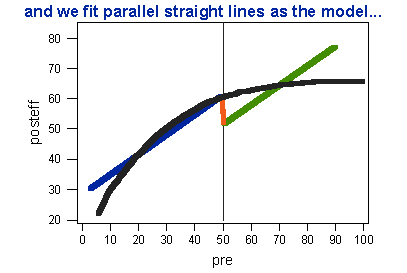Figure 2. A curvilinear relationship fit with a straight-line model.

Now, look at Figure 2. The figure shows what happens when we fit a straight-line model to the curvilinear relationship of Figure 1. In the model, we restricted the slopes of both straight lines to be the same (i.e., we did not allow for any interaction between the program and the pretest). You can see that the straight line model suggests that there is a jump at the cutoff, even though we can see that in the true function there is no discontinuity.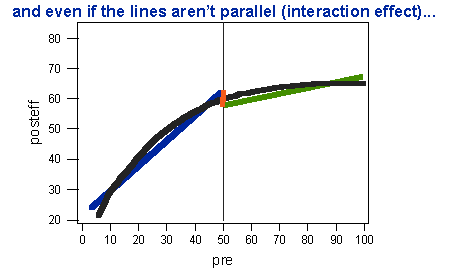Figure 3. A curvilinear relationship fit with a straight-line model with different slopes for each line (an interaction effect).

Even allowing the straight line slopes to differ doesn’t solve the problem. Figure 3 shows what happens in this case. Although the pseudo-effect in this case is smaller than when the slopes are forced to be equal, we still obtain a pseudo-effect.

The conclusion is a simple one. If the true model is curved and we fit only straight-lines, we are likely to conclude wrongly that the treatment made a difference when it did not. This is a specific instance of the more general problem of model specification.

## Model Specification

To understand the model specification issue and how it relates to the RD design, we must distinguish three types of specifications. Figure 4 shows the case where we exactly specify the true model. What does “exactly specify” mean? The top equation describes the “truth” for the data. It describes a simple straight-line pre-post relationship with a treatment effect. Notice that it includes terms for the posttest Y, the pretest X, and the dummy-coded treatment variable Z. The bottom equation shows the model that we specify in the analysis. It too includes a term for the posttest Y, the pretest X, and the dummy-coded treatment variable Z. And that’s all it includes – there are no unnecessary terms in the model that we specify. When we exactly specify the true model, we get unbiased and efficient estimates of the treatment effect.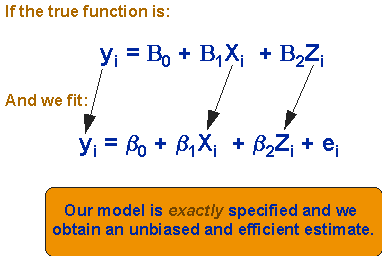Figure 4. An exactly specified model.

Now, let’s look at the situation in Figure 5. The true model is the same as in Figure 4. However, this time we specify an analytic model that includes an extra and unnecessary term. In this case, because we included all of the necessary terms, our estimate of the treatment effect will be unbiased. However, we pay a price for including unneeded terms in our analysis – the treatment effect estimate will not be efficient. What does this mean? It means that the chance that we will conclude our treatment doesn’t work when it in fact does is increased. Including an unnecessary term in the analysis is like adding unnecessary noise to the data – it makes it harder for us to see the effect of the treatment even if it’s there.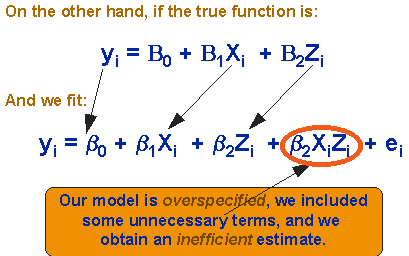Figure 5. An overspecified model.

Finally, consider the example described in Figure 6. Here, the truth is more complicated than our model. In reality, there are two terms that we did not include in our analysis. In this case, we will get a treatment effect estimate that is both biased and inefficient.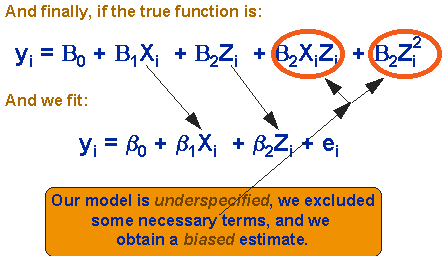Figure 6. An underspecified model.

## Analysis Strategy

Given the discussion of model misspecification, we can develop a modeling strategy that is designed, first, to guard against biased estimates and, second, to assure maximum efficiency of estimates. The best option would obviously be to specify the true model exactly. But this is often difficult to achieve in practice because the true model is often obscured by the error in the data. If we have to make a mistake – if we must misspecify the model – we would generally prefer to overspecify the true model rather than underspecify. Overspecification assures that we have included all necessary terms even at the expense of unnecessary ones. It will yield an unbiased estimate of the effect, even though it will be inefficient. Underspecification is the situation we would most like to avoid because it yields both biased and inefficient estimates.

Given this preference sequence, our general analysis strategy will be to begin by specifying a model that we are fairly certain is overspecified. The treatment effect estimate for this model is likely to be unbiased although it will be inefficient. Then, in successive analyses, gradually remove higher-order terms until the treatment effect estimate appears to differ from the initial one or until the model diagnostics (e.g., residual plots) indicate that the model fits poorly.

## Steps in the Analysis

The basic RD analysis involves five steps:

1. Transform the Pretest.

The analysis begins by subtracting the cutoff value from each pretest score, creating the modified pretest term shown in Figure 7. This is done in order to set the intercept equal to the cutoff value. How does this work? If we subtract the cutoff from every pretest value, the modified pretest will be equal to 0 where it was originally at the cutoff value. Since the intercept is by definition the y-value when x=0, what we have done is set X to 0 at the cutoff, making the cutoff the intercept point.

$$\tilde{X}_{i}=X_{i}-X_{c}$$

Figure 7. Transforming the pretest by subtracting the cutoff value.

2. Examine Relationship Visually.

There are two major things to look for in a graph of the pre-post relationship. First it is important to determine whether there is any visually discernable discontinuity in the relationship at the cutoff. The discontinuity could be a change in level vertically (main effect), a change in slope (interaction effect), or both. If it is visually clear that there is a discontinuity at the cutoff then one should not be satisfied with analytic results which indicate no program effect. However, if no discontinuity is visually apparent, it may be that variability in the data is masking an effect and one must attend carefully to the analytic results.

The second thing to look for in the bivariate relationship is the degree of polynomial which may be required as indicated by the bivariate slope of the distribution, particularly in the comparison group. A good approach is to count the number of flexion points (i.e., number of times the distribution “flexes” or “bends”) which are apparent in the distribution. If the distribution appears linear, there are no flexion points. A single flexion point could be indicative of a second (quadratic) order polynomial. This information will be used to determine the initial model which will be specified.

3. Specify Higher-Order Terms and Interactions.

Depending on the number of flexion points detected in step 2, one next creates transformations of the modified assignment variable, X. The rule of thumb here is that you go two orders of polynomial higher than was indicated by the number of flexion points. Thus, if the bivariate relationship appeared linear (i.e., there were no flexion points), one would want to create transformations up to a second-order (0 + 2) polynomial. This is shown in Figure 8. There do not appear to be any inflexion points or “bends” in the bivariate distribution of Figure 8.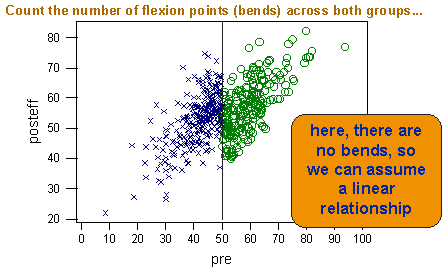Figure 8. Bivariate distribution with no flexion points.

The first order polynomial already exists in the model (X) and so one would only have to create the second-order polynomial by squaring X to obtain X2. For each transformation of X one also creates the interaction term by multiplying the polynomial by Z. In this example there would be two interaction terms: XiZi and i2Zi. Each transformation can be easily accomplished through straightforward multiplication on the computer. If there appeared to be two flexion points in the bivariate distribution, one would create transformations up to the fourth (2 + 2) power and their interactions.

Visual inspection need not be the only basis for the initial determination of the degree of polynomial which is needed. Certainly, prior experience modeling similar data should be taken into account. The rule of thumb given here implies that one should err on the side of overestimating the true polynomial function which is needed for reasons outlined above in discussing model specification. For whatever power is initially estimated from visual inspection one should construct all transformations and their interactions up to that power. Thus if the fourth power is chosen, one should construct all four terms X to X4 and their interactions.

4. Estimate Initial Model.

At this point, one is ready to begin the analysis. Any acceptable multiple regression program can be used to accomplish this on the computer. One simply regresses the posttest scores, Y, on the modified pretest X, the treatment variable Z, and all higher-order transformations and interactions created in step 3 above. The regression coefficient associated with the Z term (i.e., the group membership variable) is the estimate of the main effect of the program. If there is a vertical discontinuity at the cutoff it will be estimated by this coefficient. One can test the significance of the coefficient (or any other) by constructing a standard t-test using the standard error of the coefficient which is invariably supplied in the computer program output.

$$y_{i}=\beta_{0}+\beta_{1} \tilde{X}_{i}+\beta_{2} Z_{i}+\beta_{3} \tilde{X}_{i} Z_{i}+\beta_{4} \tilde{X}_{i}^{2}+\beta_{5} X_{i}^{2} Z_{i}+e_{i}$$

Where:

• yi = outcome score for the ith unit

• β0 = coefficient for the intercept

• β1 = linear pretest coefficient

• β2 = mean difference for treatment

• β3 = linear interaction

• β4 = quadratic pretest coefficient

• β5 = quadratic interaction

• Xi = transformed pretest

• Zi = dummy variable for treatment (0=control, 1 = treatment)

• ei = residual for the ith unit

Figure 9. The initial model for the case of no flexion points (full quadratic model specification).

If the analyst at step 3 correctly overestimated the polynomial function required to model the distribution then the estimate of the program effect will at least be unbiased. However, by including terms which may not be needed in the true model, the estimate is likely to be inefficient, that is, standard error terms will be inflated and hence the significance of the program effect may be underestimated. Nevertheless, if at this point in the analysis the coefficient is highly significant, it would be reasonable to conclude that there is a program effect. The direction of the effect is interpreted based on the sign of the coefficient and the direction of scale of the posttest. Interaction effects can also be examined. For instance, a linear interaction would be implied by a significant regression coefficient for the XZ term.

5. Refining the Model.

On the basis of the results of step 4 one might wish to attempt to remove apparently unnecessary terms and reestimate the treatment effect with greater efficiency. This is a tricky procedure and should be approached cautiously if one wishes to minimize the possibility of bias. To accomplish this one should certainly examine the output of the regression analysis in step 4 noting the degree to which the overall model fits the data, the presence of any insignificant coefficients and the pattern of residuals. A conservative way to decide how to refine the model would be to begin by examining the highest-order term in the current model and its interaction. If both coefficients are nonsignificant, and the goodness-of-fit measures and pattern of residuals indicate a good fit one might drop these two terms and reestimate the resulting model. Thus, if one estimated up to a fourth-order polynomial, and found the coefficients for X4 and X4Z were nonsignificant, these terms can be dropped and the third-order model respecified. One would repeat this procedure until: a) either of the coefficients is significant; b) the goodness-of-fit measure drops appreciably; or, c) the pattern of residuals indicates a poorly fitting model. The final model may still include unnecessary terms but there are likely to be fewer of these and, consequently, efficiency should be greater. Model specification procedures which involve dropping any term at any stage of the analysis are more dangerous and more likely to yield biased estimates because of the considerable multicolinearity which will exist between the terms in the model.

## Example Analysis

It’s easier to understand how data from a RD Design is analyzed by showing an example. The data for this example are shown in Figure 10.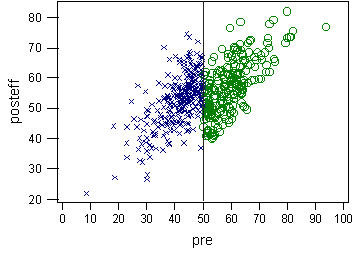Figure 10. Bivariate distribution for example RD analysis.

Several things are apparent visually. First, there is a whopping treatment effect. In fact, Figure 10 shows simulated data where the true treatment effect is 10 points. Second, both groups are well described by straight lines – there are no flexion points apparent. Thus, the initial model we’ll specify is the full quadratic one shown above in Figure 9.

The results of our initial specification are shown in Figure 11. The treatment effect estimate is the one next to the “group” variable. This initial estimate is 10.231 (SE = 1.248) – very close to the true value of 10 points. But notice that there is evidence that several of the higher-order terms are not statistically significant and may not be needed in the model. Specifically, the linear interaction term “linint” (XZ), and both the quadratic (X2) and quadratic interaction (X2Z) terms are not significant.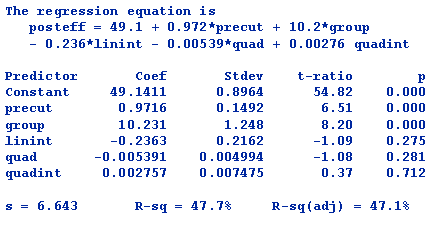Figure 11. Regression results for the full quadratic model.

Although we might be tempted (and perhaps even justified) to drop all three terms from the model, if we follow the guidelines given above in Step 5 we will begin by dropping only the two quadratic terms “quad” and “quadint”. The results for this model are shown in Figure 12.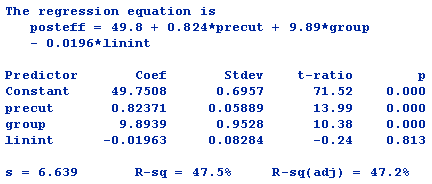Figure 12. Regression results for initial model without quadratic terms.

We can see that in this model the treatment effect estimate is now 9.89 (SE = .95). Again, this estimate is very close to the true 10-point treatment effect. Notice, however, that the standard error (SE) is smaller than it was in the original model. This is the gain in efficiency we get when we eliminate the two unneeded quadratic terms. We can also see that the linear interaction term “linint” is still nonsignificant. This term would be significant if the slopes of the lines for the two groups were different. Visual inspection shows that the slopes are the same and so it makes sense that this term is not significant.

Finally, let’s drop out the nonsignificant linear interaction term and respecify the model. These results are shown in Figure 13.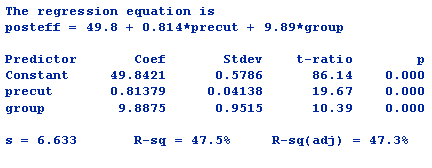Figure 13. Regression results for final model.

We see in these results that the treatment effect and SE are almost identical to the previous model and that the treatment effect estimate is an unbiased estimate of the true effect of 10 points. We can also see that all of the terms in the final model are statistically significant, suggesting that they are needed to model the data and should not be eliminated.

So, what does our model look like visually? Figure 14 shows the original bivariate distribution with the fitted regression model.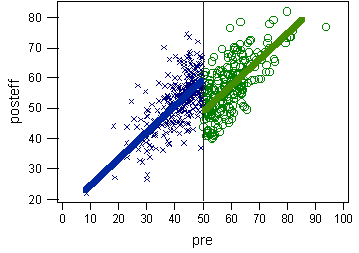Figure 14. Bivariate distribution with final regression model.

Clearly, the model fits well, both statistically and visually.